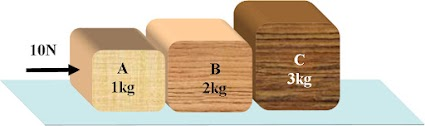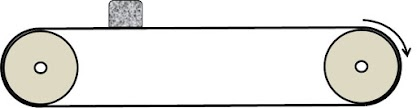Physics Quiz #1(Physics Foundation)
1. Scalar quantity has only magnitude and negave magnitude has no meaning. But work done can be negative, because:
1 point
2. Charge can have positive and negative values because:
1 point
3. Time of flight of a body projected vertically upwards without consdidering the air resistance is T and real time of flight (under the action of air resistance only) is T'. Then,
2 points
4. A body starting from rest with a constant acceleration covers 18m between t=6s to t = 8s interval. What is its acceleration?
1 point
5. A ball thrown vertically upward, has velocity approximately 22 m/s at the moment 1s before it reaches the maximum height. There is no air resistance or force of buoyancy acting on the body. It is possible if the ball has been projected from the surface of
2 points
6.A box of mass 10kg is placed on the floor of the truck. Truck starts accelerating at a rate 2m/s2. If coefficent of friction between the box and the truck-floor is 0.9. Taking the value of gravitional acceleration as 10 m/s2, the net force acting on the box as observed from the ground is:
2 points
7. An observer A notices another observer B accelerating with an acceleration 4m/s2. Choose the correct possibility:
1 point
8. Three bodies A, B and C are kept in contact on a frictionless surface as shown in the figure. Forces of same magnitude are applied on A and C successively to push the bodies.
(More than one alternative may be correct)
2 points9. A body of mass 1 kg falls from rest through 100m and hits the ground with speed 40m/s. The work done by the air resistance on the body during the fall is
1 point
10. A box is placed on a conveyer belt as shown in the figure. Coefficient of friction between the box and the belt is 0.4. Belt accelerates with acceleration 5m/s2 for 1 s and then retards with the value of retardation and stops. Distance travelled by the box duing this period in reference frame of earth is:
1 point